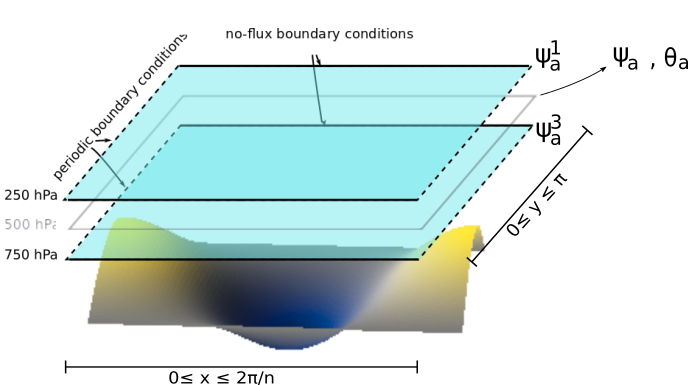# Atmospheric component

The atmospheric component is a two-layer quasi-geostrophic (QG) atmosphere in the beta-plane approximation. The atmospheric component is an extension of the QG model, first developed by [AC-CS80] and further refined by [AC-RP82] and [AC-CT87].Sketch of the atmospheric model layers with a simple orography. The domain ($$\beta$$-plane) zonal and meridional coordinates are labeled as the $$x$$ and $$y$$ variables.

The equations of motion for the atmospheric streamfunction fields $$\psi^1_\text{a}$$ at 250 hPa and $$\psi^3_\text{a}$$ at 750 hPa, and the vertical velocity $$\omega = \text{d}p/\text{d}t$$, read

$\begin{split}\frac{\partial}{\partial t} \overbrace{\left(\nabla^2 \psi^1_{\rm a}\right) }^{\text{vorticity}}+ \overbrace{J(\psi^1_{\rm a}, \nabla^2 \psi^1_{\rm a})}^{\text{horizontal advection}} + \overbrace{\beta \frac{\partial \psi^1_{\rm a}}{\partial x}}^{\beta\text{-plane approximation} \\ \text{of the Coriolis force}} & = \overbrace{-k'_d \nabla^2 (\psi^1_{\rm a}-\psi^3_{\rm a})}^{\text{friction}} + \overbrace{\frac{f_0}{\Delta p} \omega}^{\text{vertical stretching}} \nonumber \\ \frac{\partial}{\partial t} \left( \nabla^2 \psi^3_{\rm a} \right) + \, \ J(\psi^3_{\rm a}, \nabla^2 \psi^3_{\rm a}) \, \ + \qquad \beta \frac{\partial \psi^3_{\rm a}}{\partial x} \qquad & = +k'_d \nabla^2 (\psi^1_{\rm a}-\psi^3_{\rm a}) - \quad \ \frac{f_0}{\Delta p} \omega \nonumber \\\end{split}$

where $$\nabla^2$$ is the horizontal Laplacian. The Coriolis parameter $$f$$ is linearized around a value $$f_0$$ (f0) evaluated at latitude $$\phi_0$$ (phi0_npi), $$f = f_0 + \beta y$$, with $$\beta=\text{d}f/\text{d}y$$ (beta). The parameter $$k'_d$$ (kdp) quantify the friction between the two atmospheric layers, and $$\Delta p = 500$$ hPa (deltap) is the pressure difference between the atmospheric layers. Finally, $$J$$ is the Jacobian $$J(S, G) = \partial_x S\, \partial_y G - \partial_y S\, \partial_x G$$.